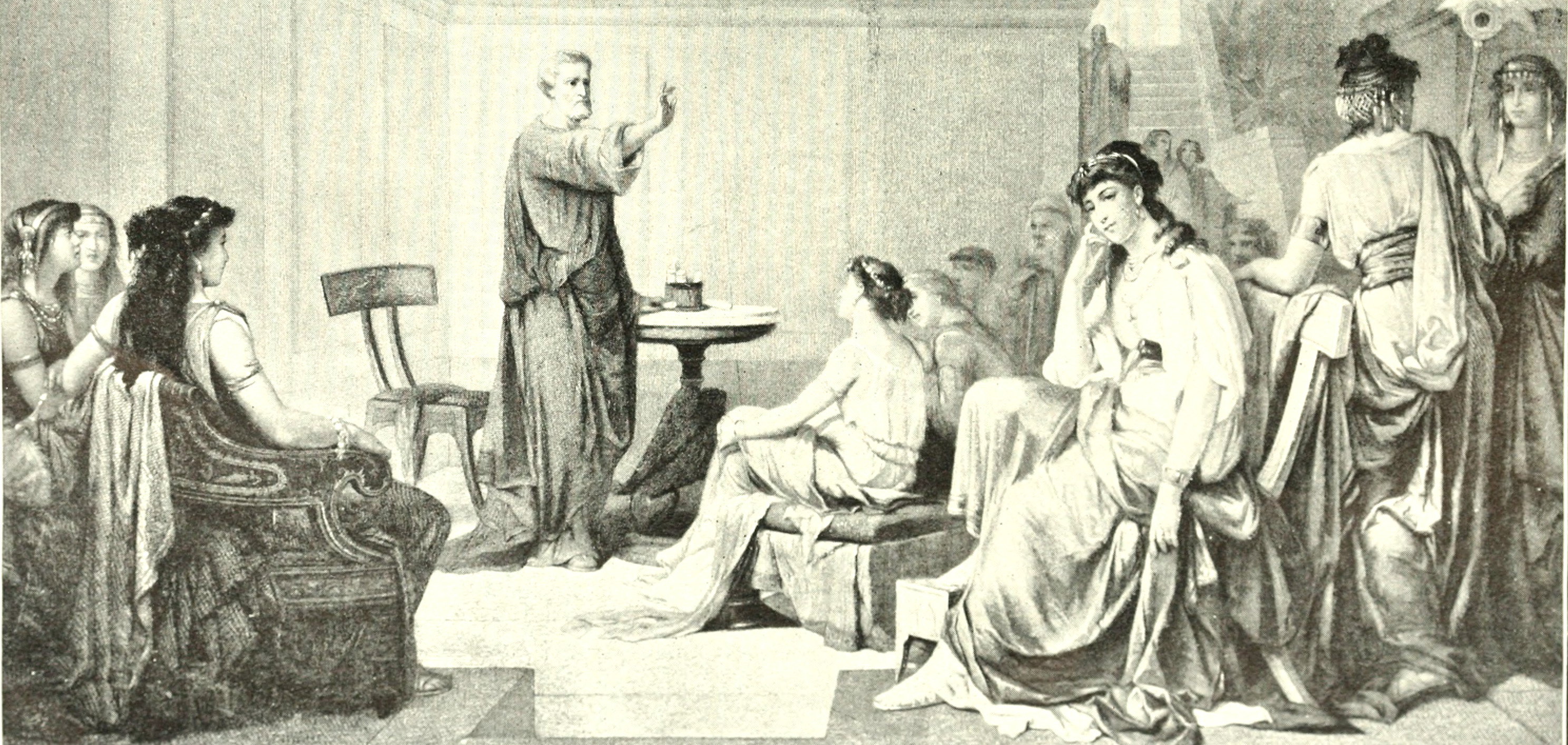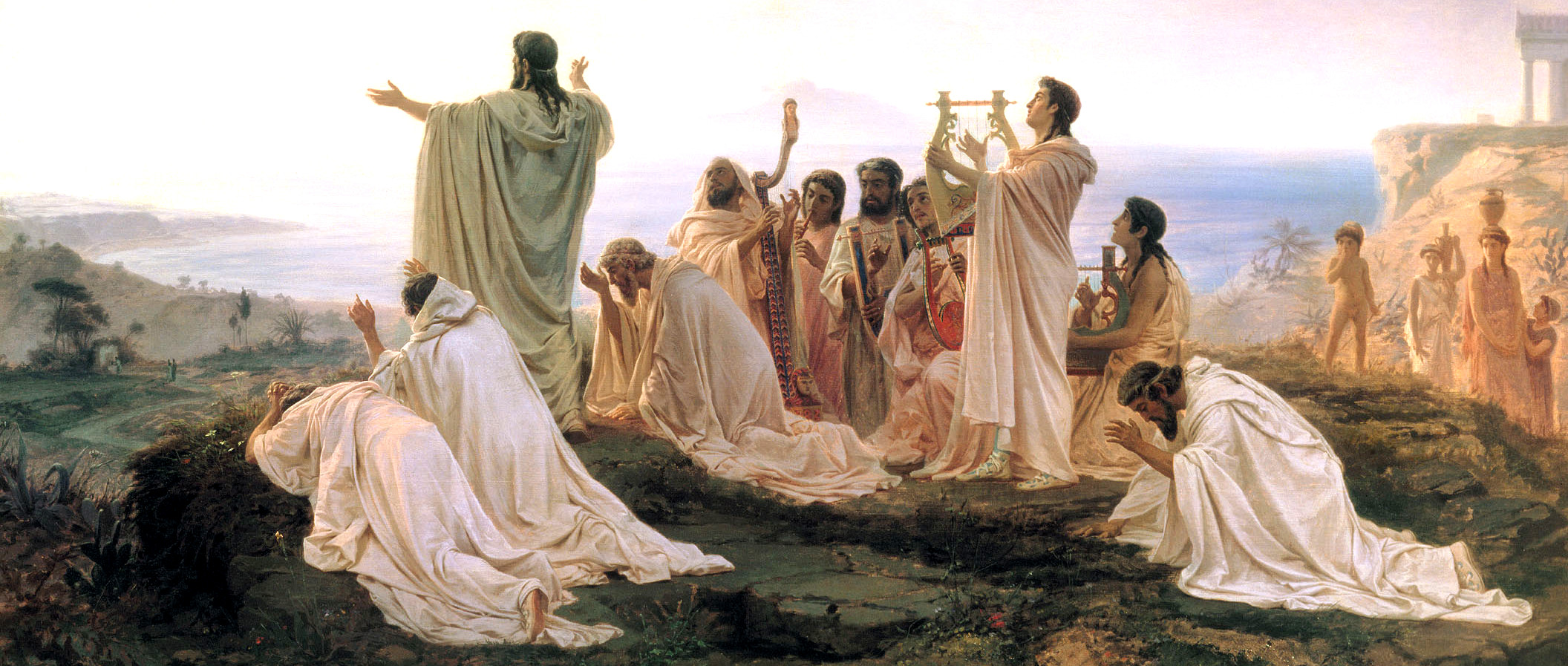# Pythagoras

Pythagoras of Samos lived from 570 to 495 BC. The Pythagorean theorem applies in any vector space with inner product assigning to two vectors a number. The theorem is the relation a2+b2=c2, where a=|v|, b=|w| and c=|v-w| are lengths of a right angle triangle formed by the points A, B=A+v, C=A+w where AB=v, AC=w, CB=v-w are the vectors connecting the points. The right angle assumption is written mathematically in the form v.w=0. The length is defined as |v| = (v.v)1/2. The theorem then follows as c2 = (v-w).(v-w) = v.v - 2 v.w + w.w = a2 + b2. This theorem is so important that it can be considered the ``fundamental theorem of geometry".Pythagoras teaching: From "The story of the greatest nations", (1910) From ArchivThe Pythagoreans: Fyodor Bronnikov, (1827-1902)